# How Many Typos?#

When I started work at Brilliant a couple of weeks ago, I learned that one of my new colleagues, Michelle McSweeney, just published a book called OK, which is all about the word OK.

As we discussed the joys and miseries of publishing, Michelle mentioned that she had found a typo in the book after publication. So naturally I took it as a challenge to find the typo. While I was searching, I enjoyed the book very much. If you are interested in etymology, linguistics, and history, I recommend it!

As it turned out, I found exactly one typo. When I told Michelle, she asked me nervously which page it was on. Page 17. She looked disappointed – that was not the same typo she found.

Now, for people who like Bayesian statistics, this scenario raises some questions:

1. After our conversation, how many additional typos should we expect there to be?

2. If she and I had found the same typo, instead of different ones, how many typos would we expect?

As it happens, I used a similar scenario as an example in Think Bayes. This notebook is based on the code I presented there. If there’s anything here that’s not clear, you could read the chapter for more details.

## A Warm-up Problem#

Starting with a simple version of the problem, let’s assume that we know `p0` and `p1`, which are the probabilities, respectively, that the first and second readers find any given typo.

For example, let’s assume that Michelle has a 66% chance of finding a typo and I have a 50% chance.

```p0, p1 = 0.66, 0.5
```

With these assumptions, we can compute an array that contains (in order) the probability that neither of us find a typo, the probability that only the second reader does, the probability that the first reader does, and the probability that we both do.

```def compute_probs(p0, p1):
"""Computes the probability for each of 4 categories."""
q0 = 1-p0
q1 = 1-p1
return [q0*q1, q0*p1, p0*q1, p0*p1]
```
```y = compute_probs(p0, p1)
y
```
```[0.16999999999999998, 0.16999999999999998, 0.33, 0.33]
```

With the assumed probabilities, there is a 33% chance that we both find a typo and a 17% chance that neither of us do.

## The Prior#

Next we need to choose a prior for the total number of typos. I’ll use a Poisson distribution, which is a reasonable default for values that are counts. And I’ll set the mean to 2, which means that before either of us read the book, we would have expected about two typos.

```from utils import make_poisson_pmf

qs = np.arange(0, 11)
lam = 2
prior_N = make_poisson_pmf(lam, qs)
prior_N.index.name = 'N'
prior_N.mean()
```
```1.9999236196641172
```

Here’s what the prior looks like.

Hide code cell source
```prior_N.plot(color='C7')

decorate(xlabel='Number of typos (N)',
ylabel='PMF',
title='Prior distribution')
```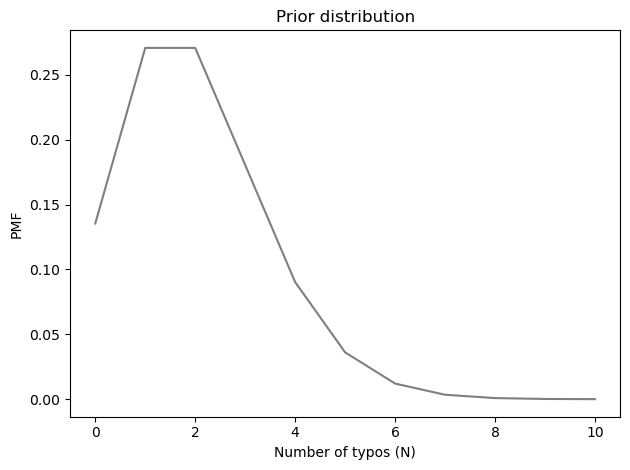## The Update (Simple Version)#

To represent the data, I’ll create these variables:

• `k10`: the number of typos found by the first reader but not the second

• `k01`: the number of typos found by the second reader but not the first

• `k11`: the number of typos found by both readers

```k10 = 1
k01 = 1
k11 = 0
```

I’ll put the data in an array, with 0 as a place-keeper for the unknown value `k00`, which is the number of typos neither of us found.

```data = np.array([0, k01, k10, k11])
```

Now we can use the multinomial distribution to compute the likelihood of the data for each hypothetical value of `N` (assuming for now that the probabilities in `y` are known).

```from scipy.stats import multinomial

likelihood = prior_N.copy()
observed = data.sum()
x = data.copy()

for N in prior_N.qs:
x = N - observed
likelihood[N] = multinomial.pmf(x, N, y)
```

We can compute the posterior in the usual way.

```posterior_N = prior_N * likelihood
posterior_N.normalize()
```
```0.0426675416210306
```

And here’s what it looks like.

Hide code cell source
```prior_N.plot(color='C7', alpha=0.4, label='prior')
posterior_N.plot(color='C4', label='posterior')

decorate(xlabel='Number of typos (N)',
ylabel='PMF',
title='Prior distribution')
```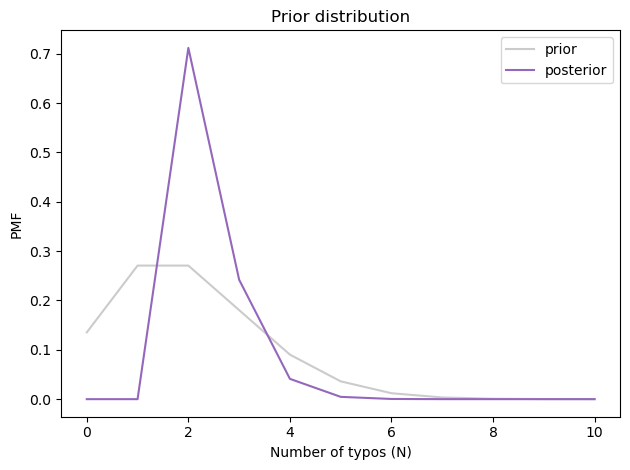The most likely value is 2, and the posterior mean is about 2.3, just a little higher than the prior mean.

Hide code cell content
```print(posterior_N.mean(),
posterior_N.credible_interval(0.9))
```
```2.3399999989281604 [2. 3.]
```

But remember that this is based on the assumption that we know `p0` and `p1`. In reality, they are unknown, but we can estimate them from the data.

## Three-Parameter Model#

What we need is a model with three parameters: `N`, `p0`, and `p1`. We’ll use `prior_N` again for the prior distribution of `N`, and here are the priors for `p0` and `p1`:

```from empiricaldist import Pmf
from scipy.stats import beta as beta_dist

def make_beta(qs, alpha, beta, name=""):
ps = beta_dist.pdf(qs, alpha, beta)
pmf = Pmf(ps, qs)
pmf.normalize()
pmf.index.name = name
return pmf
```
```qs = np.linspace(0, 1, num=51)
prior_p0 = make_beta(qs, 3, 2, name='p0')
prior_p1 = make_beta(qs, 2, 2, name='p1')
```
```prior_p0.plot()
prior_p1.plot()

decorate(xlabel='Probability of finding a typo',
ylabel='PMF',
title='Prior distributions')
```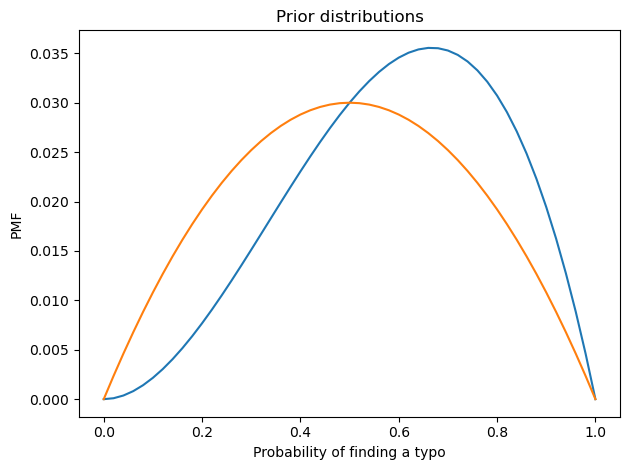I used beta distributions to construct weakly informative priors for `p0` and `p1`, with means `0.66` and `0.5` respectively.

## The Joint Prior#

Now we have to assemble the marginal priors into a joint distribution. I’ll start by putting the first two into a `DataFrame`.

```from utils import make_joint

joint2 = make_joint(prior_p0, prior_N)
joint2.shape
```
```(11, 51)
```

Now I’ll stack them and put the result in a `Pmf`.

```joint2_pmf = Pmf(joint2.stack())
```
probs
N p0
0 0.00 0.000000
0.02 0.000013
0.04 0.000050

We can use `make_joint` again to add in the third parameter.

```joint3 = make_joint(prior_p1, joint2_pmf)
joint3.shape
```
```(561, 51)
```

The result is a `DataFrame` with values of `N` and `p0` in a `MultiIndex` that goes down the rows and values of `p1` in an index that goes across the columns.

```joint3.head(3)
```
p1 0.00 0.02 0.04 0.06 0.08 0.10 0.12 0.14 0.16 0.18 ... 0.82 0.84 0.86 0.88 0.90 0.92 0.94 0.96 0.98 1.00
N p0
0 0.00 0.0 0.000000e+00 0.000000e+00 0.000000e+00 0.000000e+00 0.000000e+00 0.000000e+00 0.000000e+00 0.000000e+00 0.000000e+00 ... 0.000000e+00 0.000000e+00 0.000000e+00 0.000000e+00 0.000000e+00 0.000000e+00 0.000000e+00 0.000000e+00 0.000000e+00 0.0
0.02 0.0 2.997069e-08 5.871809e-08 8.624220e-08 1.125430e-07 1.376205e-07 1.614748e-07 1.841057e-07 2.055133e-07 2.256977e-07 ... 2.256977e-07 2.055133e-07 1.841057e-07 1.614748e-07 1.376205e-07 1.125430e-07 8.624220e-08 5.871809e-08 2.997069e-08 0.0
0.04 0.0 1.174362e-07 2.300791e-07 3.379286e-07 4.409848e-07 5.392478e-07 6.327174e-07 7.213937e-07 8.052767e-07 8.843664e-07 ... 8.843664e-07 8.052767e-07 7.213937e-07 6.327174e-07 5.392478e-07 4.409848e-07 3.379286e-07 2.300791e-07 1.174362e-07 0.0

3 rows × 51 columns

Now I’ll apply `stack` again:

```joint3_pmf = Pmf(joint3.stack())
```
probs
N p0 p1
0 0.0 0.00 0.0
0.02 0.0
0.04 0.0

The result is a `Pmf` with a three-column `MultiIndex` containing all possible triplets of parameters.

The number of rows is the product of the number of values in all three priors:

```joint3_pmf.shape
```
```(28611,)
```

That’s still small enough to be practical, but it will take longer to compute the likelihoods than in the previous example.

## The Update (Three-Parameter Version)#

Here’s the loop that computes the likelihoods. It’s similar to the one in the previous section, except that `y` is no longer constant; we compute it each time through the loop from hypothetical values of `p0` and `p1`.

```likelihood = joint3_pmf.copy()
observed = data.sum()
x = data.copy()

for N, p0, p1 in joint3_pmf.index:
x = N - observed
y = compute_probs(p0, p1)
likelihood[N, p0, p1] = multinomial.pmf(x, N, y)
```
```/home/downey/miniconda3/envs/ThinkBayes2/lib/python3.10/site-packages/scipy/stats/_multivariate.py:3190: RuntimeWarning: invalid value encountered in subtract
return gammaln(n+1) + np.sum(xlogy(x, p) - gammaln(x+1), axis=-1)
```

We can compute the posterior in the usual way.

```posterior_pmf = joint3_pmf * likelihood
posterior_pmf.normalize()
```
```0.03437554251769621
```

Here’s the posterior marginal distribution `N`.

```posterior_N = posterior_pmf.marginal(0)
```
```prior_N.plot(color='C7', alpha=0.4, label='prior')
posterior_N.plot(color='C4', label='posterior')

decorate(xlabel='Number of bugs (N)',
ylabel='PDF',
title='Posterior marginal distributions of N')
```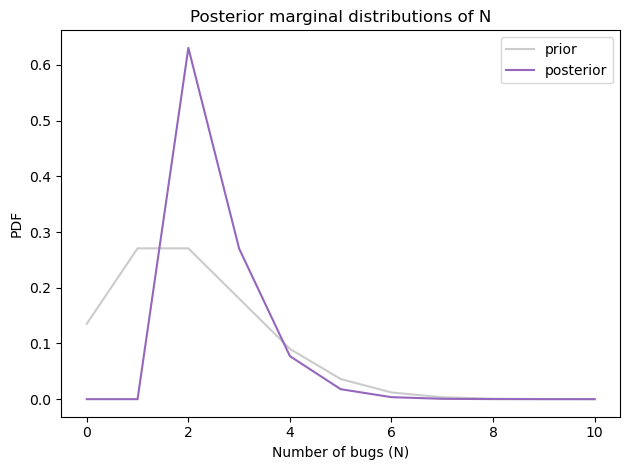To compute the probability that there is at least one undiscovered typo, we can convert the posterior distribution to a survival function (complementary CDF):

```posterior_N.make_surv()[observed]
```
```0.369576444173048
```

The probability is about 37% that there’s another typo – so that’s good news!

The posterior mean is about 2.5, which is a little higher than what we got with the simple model.

```posterior_N.mean()
```
```2.4963989774563107
```

Apparently our uncertainty about `p0` and `p1` leaves open the possibility that there are more typos and we are not very good at finding them. Here’s what the posterior distributions look like for `p0` and `p1`.

Hide code cell source
```posterior_p1 = posterior_pmf.marginal(1)
posterior_p2 = posterior_pmf.marginal(2)

posterior_p1.plot(label='p1')
posterior_p2.plot(label='p2')

decorate(xlabel='Probability of finding a bug',
ylabel='PDF',
title='Posterior marginal distributions of p1 and p2')
```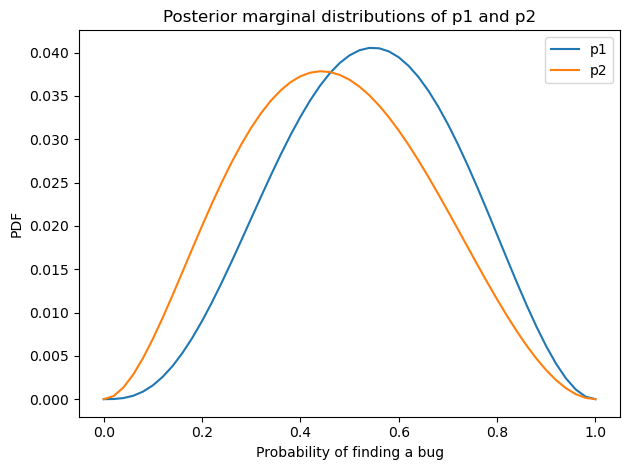With so little data, the posterior distributions are still quite wide, but the posterior means are a little smaller than the priors.

```posterior_p1.mean(), posterior_p1.credible_interval(0.9)
```
```(0.5383375553393667, array([0.24, 0.82]))
```
```posterior_p2.mean(), posterior_p2.credible_interval(0.9)
```
```(0.46718395159883724, array([0.16, 0.78]))
```

The fact that Michelle and I found only two typos is weak evidence that we are not as good at finding them as the priors implied.

At this point, we’ve answered the first question: given that Michelle and I found different bugs, the expected value for the number of remaining typos is about 0.5.

In the counterfactual case, if we had found the same typo, we would represent the data like this:

```k10 = 0
k01 = 0
k11 = 1
```

If we run the analysis with this data, we find:

• The expected number of remaining typos is about 0.3 (compared to 0.5),

• there would be only a 25% chance that there is at least one undiscovered typo, and

• we would conclude that Michelle and I are slightly better at finding typos.

This notebook is based on this chapter of Think Bayes, Second Edition.